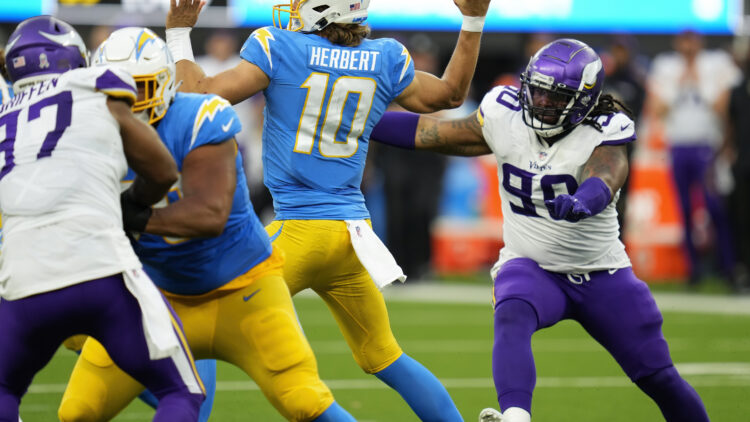# NFL’s Top QBs after Week 12: PFF, QBR, Passer Rating Formula#### The following scores are “good” through December 2, 2021..

There is a healthy fight within the stat community on which quarterback metric is most coherent. Passer rating is bashed as too antiquated. When used to compare quarterbacks from eras of yesteryear, passer rating is indeed skewed and inflated. But touchdowns, passing yards, interception, and completion percentage will never be wholly irrelevant.

ESPN’s QBR is said to factor in more intangible-type ratings. It can be interpreted as a more detailed and comprehensive version of passer rating and then some.

Pro Football Focus uses its grading system to assess the x’s and o’s of each game and pump out a yearly score.

The following scores and rankings are weighted. Passer rating accounts for 20% of the score, QBR at 40%, and PFF at 40%. Skill position players and players with menial pass attempts were excluded.

## 34. Zach Wilson (NYJ), 44.5

PFF = 56.3, QBR = 23.5, P-Rating = 62.9

## 33. Justin Fields (CHI), 49.8

PFF = 64.2, QBR = 25.9, P-Rating = 69.0

## 32. Trevor Lawrence (JAX), 51.9

PFF = 59.8, QBR = 33.9, P-Rating = 72.3

## 31. Davis Mills (HOU), 51.9

PFF = 59.3, QBR = 30.5, P-Rating = 80.2

## 30. Jared Goff (DET), 53.3

PFF = 60.6, QBR = 29.3, P-Rating = 87.0

## 29. Sam Darnold (CAR), 53.5

PFF = 62.6, QBR = 35.6, P-Rating = 71.3

## 28. Trevor Siemian (NO), 56.2

PFF = 65.1, QBR = 32.5, P-Rating = 86.0

## 27. Ben Roethlisberger (PIT), 57.5

PFF = 56.4, QBR = 43.1, P-Rating = 88.9

## 26. Baker Mayfield (CLE), 59.2

PFF = 65.3, QBR = 37.9, P-Rating = 89.9

## 25. Tyrod Taylor (HOU), 60.2

PFF = 62.6, QBR = 46.8, P-Rating = 82.4

## 24. Daniel Jones (NYG), 61.9

PFF = 71.6, QBR = 40.9, P-Rating = 84.8

## 23. Taylor Heinicke (WAS), 63.7

PFF = 64.8, QBR = 48.8, P-Rating = 91.3

## 22. Jacoby Brissett (MIA), 66.2

PFF = 73.5, QBR = 50.5, P-Rating = 78.1

## 21. Lamar Jackson (BAL), 67.3

PFF = 73.5, QBR = 51.1, P-Rating = 87.5

## 20. Jalen Hurts (PHI), 67.9

PFF = 80.0, QBR = 48.0, P-Rating = 83.9

## 19. Russell Wilson (SEA), 68.5

PFF = 73.9, QBR = 46.2, P-Rating = 102.5

## 18. Matt Ryan (ATL), 69.2

PFF = 78.0, QBR = 50.5, P-Rating = 89.1

## 17. Carson Wentz (IND), 70.8

PFF = 72.3, QBR = 56.7, P-Rating = 96.0

## 16. Ryan Tannehill (TEN), 70.8

PFF = 81.4, QBR = 53.4, P-Rating = 84.7

## 15. Teddy Bridgewater (DEN), 71.3

PFF = 78.7, QBR = 50.1, P-Rating = 98.9

## 14. Patrick Mahomes (KC), 71.3

PFF = 73.7, QBR = 50.1, P-Rating = 94.7

## 13. Tua Tagovailoa (MIA), 71.4

PFF = 73.5, QBR = 57.7, P-Rating = 94.7

## 12. Jimmy Garoppolo (SF), 72.1

PFF = 72.3, QBR = 58.6, P-Rating = 99.1

## 11. Derek Carr (LV), 73.9

PFF = 79.1, QBR = 57.2, P-Rating = 97.3

## 10. Mac Jones (NE), 74.0

PFF = 83.9, QBR = 52.6, P-Rating = 97.1

## 9. Dak Prescott (DAL), 74.8

PFF = 84.4, QBR = 50.6, P-Rating = 104.2

## 8. Joe Burrow (CIN), 75.3

PFF = 86.2, QBR = 51.3, P-Rating = 101.6

## 7. Josh Allen (BUF), 76.8

PFF = 82.0, QBR = 60.6, P-Rating = 99.1

## 6. Matthew Stafford (LAR), 77.8

PFF = 76.2, QBR = 65.8, P-Rating = 105.3

## 5. Kirk Cousins (MIN), 79.2

PFF = 88.2, QBR = 57.0, P-Rating = 105.2

## 4. Justin Herbert (LAC), 79.9

PFF = 85.8, QBR = 65.9, P-Rating = 96.5

## 3. Aaron Rodgers (GB), 80.1

PFF = 82.7, QBR = 64.9, P-Rating = 105.5

## 2. Kyler Murray (ARI), 81.7

PFF = 85.6, QBR = 63.5, P-Rating = 110.4

## 1. Tom Brady (TB), 82.6

PFF = 91.5, QBR = 63.6, P-Rating = 103.1

Dustin Baker is a political scientist who graduated from the University of Minnesota in 2007. He hosts a podcast with Bryant McKinnie, which airs every Wednesday with Raun Sawh and Sally from Minneapolis. His Viking fandom dates back to 1996. Listed guilty pleasures: Peanut Butter Ice Cream, ‘The Sopranos,’ and The Doors (the band).

Share: